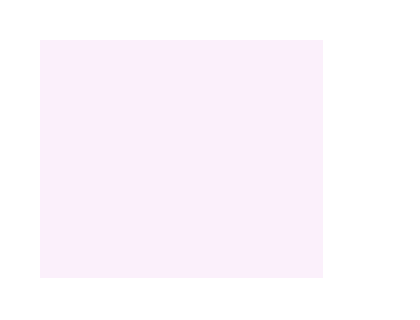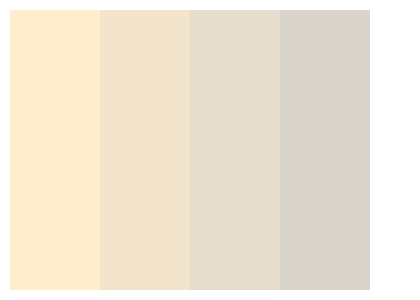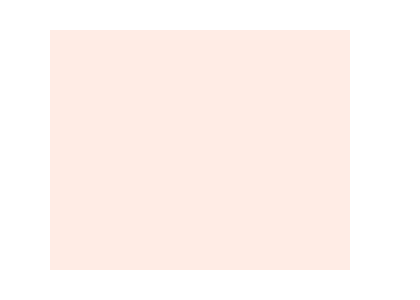# Math.round() / Math.floor() / Math.ceil()

## Overview

The `Math.round()` function accepts a number and returns its value rounded to the nearest integer:

``Math.round(x);``

The `Math.floor()` and `Math.ceil()` functions return the value of a number rounded down to the nearest integer and rounded up, respectively:

``````Math.floor(x);
Math.ceil(x);``````

The `Math.round()`, `Math.floor()`, and `Math.ceil()` functions are methods of the `Math` object, which has a set of functions that other programmers have defined for us to use. Here is a full list of available

## Round a Number

In this example, we use the `Math.round()`, `Math.floor()`, and `Math.ceil()` functions to round a number assigned to the variable `x`.

``````var x = 8.3962; // Assign the number to round

var a = Math.round(x);
var b = Math.floor(x);
var c = Math.ceil(x);``````

Change the value assigned to the variable `x` to see how the `Math.round()`, `Math.floor()`, and `Math.ceil()` functions work. You can also assign the variable an arithmetic expression, such as `17 / 3` .

Quick Reference:

Message Log

## Round the Dimensions of a Rectangle

In this example, we use the `Math.floor()` and `Math.ceil()` functions to round the dimensions of a rectangle.

We start by assigning two decimal numbers to the variables `a` and `b`.

``````var a = 183.67;
var b = 107.44;``````

Then, we use the `Math.floor()` function to round `a` down to the nearest integer for the width of the rectangle and the `Math.ceil()` function to round `b` up to the nearest integer for the height.

``````var w = Math.floor(a);
var h = Math.ceil(b);``````

When drawing the rectangle using the `context.fillRect()` method, the rectangle has a width of 183 and a height of 108. Change the values assigned to the variables `a` and `b` to see how the dimensions of the rectangle change.

Quick Reference:

Message Log

## Challenge 1

Calculate the width of the rectangle by rounding the value of the variable `a` up to the nearest integer and calculate the height by rounding the value of the variable `b` also up to the nearest integer.

Message Log
##### Canvas (your drawing will display here)## Calculate a Color

When describing a color, RGB values must be whole numbers between 0 and 255.

In this example, we describe one color using RGB values assigned to the variables `r1`, `g1`, and `b1` and a second color using RGB values assigned to the variables `r2`, `g2`, and `b2`.

``````var r1 = 255; // RGB values for color 1
var g1 = 0;
var b1 = 0;

var r2 = 0; // RGB values for color 2
var g2 = 102;
var b2 = 255;``````

We then mix the two colors to create three in-between colors. To create a color that is 75% color 1 and 25% color 2, we multiply the RGB values of color 1 by 0.75 and the RGB values of color 2 by 0.25 and add them together. In case some of the calculated RGB values for the new colors are decimals and not whole numbers, we use the `Math.round()` function to round them.

The expression to calculate the red component of a color that is 75% color 1 and 25% color 2 looks like this:

``Math.round(0.75 * r1 + 0.25 * r2) // Red component of the mixed color``

Finally, we draw five rectangles. The rectangle on the left is filled with color 1. The rectangle on the right is filled with color 2. The three rectangles in the middle are filled with the three in-between colors. Change the RGB values for color 1 and color 2 to see what happens.

Quick Reference:

Message Log

## Challenge 2

The program below draws four rectangles and fills them with the color from the RGB values assigned to the variables `r`, `g`, and `b`.

Update the program so the second rectangle is filled with a color created by multiplying the RGB values in the variables `r`, `g`, and`b`by 0.75; the third rectangle is filled with a color created by multiplying the RGB values by 0.5; and the fourth rectangle is filled with a color created by multiplying the RGB values by 0.25. Make sure to round the calculated RGB values for the new colors so they are whole numbers.

Message Log
##### Canvas (your drawing will display here)## Snap a Rectangle to a Grid

In this example, we use the `Math.round()` function to snap a rectangle to a grid.

We are registering an anonymous function to listen for `mousemove` events on the canvas. If the mouse is moved over the canvas, a `mousemove` event is generated and passed into the anonymous function, where it is then stored in the parameter `e`.

``````canvas.addEventListener('mousemove', function(e) {

// Code block

});``````

As the mouse moves over the canvas, we can access the mouse's coordinates on the canvas through the `mousemove` event's `layerX` and `layerY` properties. By setting the width of the rectangle to the value of `layerX` and the height to the value of `layerY`, we can resize the rectangle so it's bottom left corner moves with the mouse. However, we don't want the size of the rectangle to change freely as the mouse moves. We want the rectangle to snap to the grid below.

The lines on the grid are 40 pixels apart. So, to keep the rectangle aligned to the grid, the dimensions of the rectangle must be multiples of 40. We are using the `Math.round()` function to round the width and height of the rectangle to the nearest multiple of 40:

``````canvas.addEventListener('mousemove', function(e) {
var w = 40 * Math.round(e.layerX / 40);
var h = 40 * Math.round(e.layerY / 40);
});``````

Let's say the mouse's coordinates on the canvas are (190, 85). If we divide 190 by 40 and round the answer, we get 5. This means 40 goes into 190 about 5 times. Multiplying 40 by 5 then gives us 200, a multiple of 40, for the width of the rectangle. If we divide 85 by 40 and round the answer, we get 2. This means 40 goes into 85 about 2 times. Multiplying 40 by 2 then gives us 80, another multiple of 40, for the height of the rectangle. Because the dimensions of the rectangle are multiples of 40, it snaps to the grid below.

Quick Reference:

Message Log

## Challenge 3

Calculate the width of the rectangle by rounding the value of the variable `a` to the nearest multiple of 50 and calculate the height by rounding the value of the variable `b` to the nearest multiple of 30.